## Electrical Engineering Basics Objective Questions with Answers

 Electrostatics is a branch of electricity concerned with
(a) Energy flowing across a gap between conductors
(b) Charges at rest
(c) Charges in motion
(d) Energy in the form of charges

 Four 2 μF capacitors are connected in series. The equivalent capacitance is
(a) 8 μF
(b) 0.5 μF
(c) 2 μF
(d) 6 μF

 State which of the following is false.
The capacitance of a capacitor
(a) Is proportional to the cross-sectional area of the plates
(b) Is proportional to the distance between the plates
(c) Depends on the number of plates
(d) Is proportional to the relative permittivity of the dielectric

 The capacitance of a capacitor is the ratio
(a) Charge to potential difference between plates
(b) Potential difference between plates to plate spacing
(c) Potential difference between plates to thickness of dielectric
(d) Potential difference between plates to charge

 Which of the following statement is false?
(a) An air capacitor is normally a variable type
(b) A paper capacitor generally has a shorter service life than most other types of
capacitor
(c) An electrolytic capacitor must be used only on a.c. supplies
(d) Plastic capacitors generally operate satisfactorily under conditions of high temperature

 The potential difference across a 10 μF capacitor to charge it with 10mC is
(a) 10V
(b) 1 kV
(c) 1V
(d) 10V

 The energy stored in a 10μF capacitor when charged to 500V is
(a) 1.25 mJ
(b) 0.025 μJ
(c) 1.25 J
(d) 1.25 C

 The capacitance of a variable air capacitor is at maximum when
(a) The movable plates half overlap the fixed plates
(b) The movable plates are most widely separated from the fixed plates
(c) Both sets of plates are exactly meshed
(d) The movable plates are closer to one side of the fixed plate than to the other

 The unit of magnetic flux density is the:
(a) Weber
(b) Weber per metre
(c) Ampere per metre
(d) Tesla

 The charge on a 10 pF capacitor when the voltage applied to it is 10 kV is
(a) 100 μC
(b) 0.1 C
(c) 0.1 μC
(d) 0.01 μC

 Four 2 μF capacitors are connected in parallel. The equivalent capacitance is
(a) 8 μF
(b) 0.5 μF
(c) 2 μF
(d) 6 μF

 In a series a.c. circuit the voltage across a pure inductance is 12V and the voltage across a pure resistance is 5V. The supply voltage is
(a) 13V
(b) 17V
(c) 7V
(d) 2.4V

 Inductive reactance results in a current that
(a) Leads the voltage by 90deg
(b) Is in phase with the voltage
(c) Leads the voltage by π rad
(d) Lags the voltage by π/2 rad

 A 10Ω resistor is connected in parallel with a 15 Ω resistor and the combination in series with a 12 Ω resistor. The equivalent resistance of the circuit is:
(a) 37Ω
(b) 18 Ω
(c) 27 Ω
(d) 4 Ω

 The equivalent resistance when a resistor of (1/3)Ω is connected in parallel with a (1/4)Ω resistance is:
(a) 1/7 Ω
(b) 7Ω
(c) 1/12 Ω
(d) 3/4 Ω

The solutions with explanations for the problems are given in the link: Part-17 Problems and solutions
Thanks for reading.. Please leave your comments below...
7:56 AM

#### 26 comments:

1.best one objective type questions for remined your brain.

2.Plz chk question no.10

3.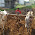Give me explanation. It ll b helpful

1.Explanations are added.... thanks for your comments

4.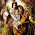Give some more basic questions from electrical design,power system

5.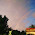Plz Give the Explanation for Question no 6,10,12,14,&15
It Will be help to me

1.Explanations are added.... thanks for your comments

6.Check Qn. 5

1.The correct answer is C... Thanks for your comment.... Electrolytic cap must always be used in DC....

2.Please to be edited......

7.Wrongly answered

8.Check the 7th sum...
Energy E = C * V²
Answer is 2.5 joule

1.2.9.Sorry it's E = 1/2 * C * V²
Right ans is 1.25 joule
Apologize...

10.We want more questions....

11.Que 15 right ans. Is 7 ohms

1.Explanations are added.... check the answers

12.HAGRU QUESTIONS

13.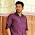Need explenation for Q. No. 12

1.Explanations are added.... thanks for your comments

14.Need explanation For q. No12

15.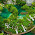when we deal with a.c circuits its always
vector sum not a arthematic sum ....so here phase angle between resistor and inductor is 90....use parallogram law to find resultant voltage

16.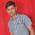question 5 answer is C please check

1.The correct answer is C... Thanks for your comment.... Electrolytic cap must always be used in DC....

17.An electrolytic capacitor must be used only on d.c. supplies